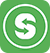4638 просмотров На чтение: 2 мин# Warehouse accounting method (how the cost is calculated)

If you receive goods at different cost prices, you can choose one of the warehouse accounting methods (at the last cost price or average cost price)
• In the Admin panel, go to Settings – Trade points, and select a trade points
• In the window that opens, edit the Inventory accounting method – select the method that suits you:
• the last cost price;
• average cost price.
• Click Save

### Average cost price method

• This method is the easiest to account for. The average cost of goods is determined by the following formula:

Average cost of goods = (cost of goods at the beginning of the month + cost of goods received per month) / (quantity of goods at the beginning of the month + quantity of goods received per month)

Calculation example using the average cost method:

• There were 20 packs of cookies left in the store at a purchase price of \$ 15. During the month, another 16 packs of cookies were delivered in two batches – 8 \$ 28 and 8 \$ 20. We calculate the average cost.

Product cost at the beginning of the month: 20 X 15 = 300
The cost of the 1st new delivery of the Goods: 8 X 28 = 224
The cost of the 2nd new delivery of the Goods: 8 X 20 = 160
Average Product Price: (300 + 224 + 160): (20 + 16) = 19

• At this average cost, the written-off goods will be considered and the profit will be calculated. For example, if a pack of cookies sells for \$ 40, and 250 packs of cookies were sold in a month, the profit for that particular cookie will be calculated as follows:

250 X 40 – 250 X 19 = 5250 \$

### Done!